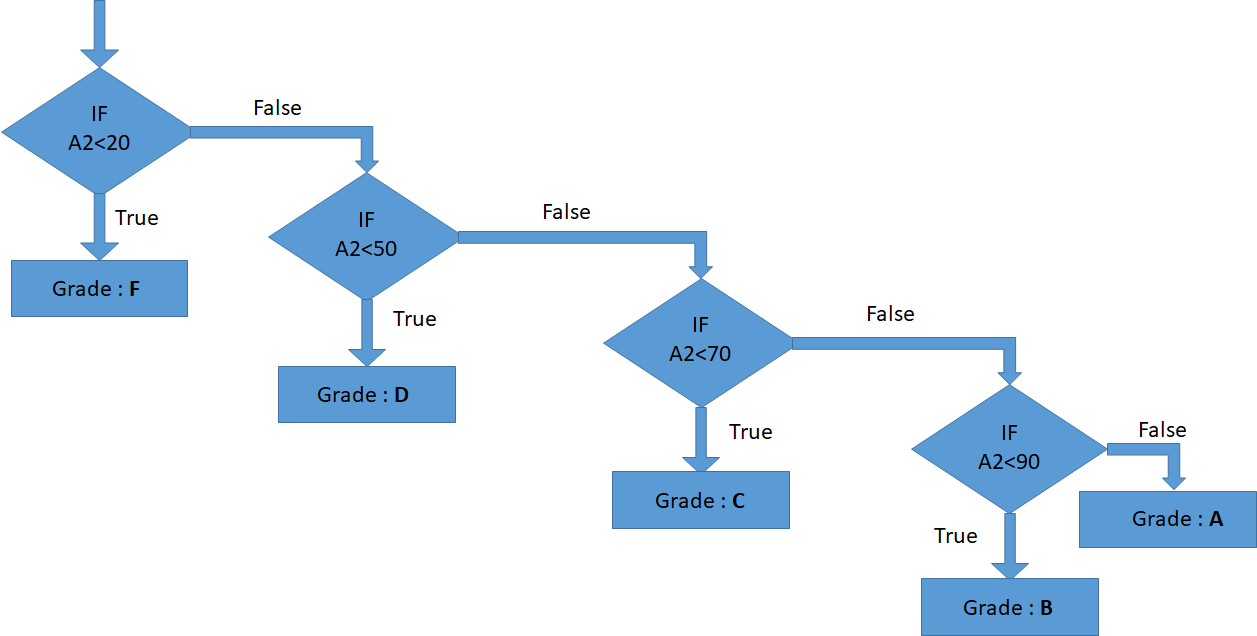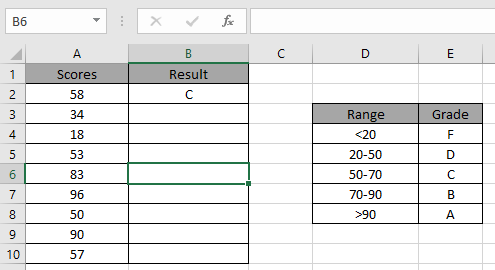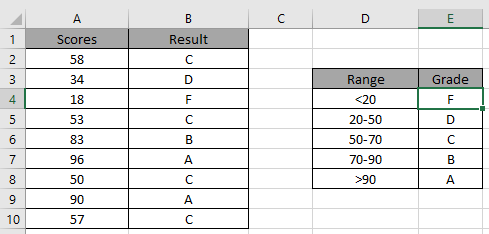# How to Use Nested IF Function in Excel

IF function is used for logic_test and returns value on the basis of the result of the logic_test.
Syntax:

=IF (logical_test, [value_if_true], [value_if_false])

Nested IF is a iteration of IF function. It runs multiple logic_test in one formula. IFS function is built in function use instead for nested IF function in version above Excel 2016..

Let’s understand this function using it an example.We need to find the Grade for the corresponding Scores.

Use the Formula

=IF(A2 < 20,"F",IF(A2 < 50,"D",IF(A2 < 70,"C",IF(A2 < 90,"B","A"))))

Explanation:The flow chart above explains the nested IF logic.Applying the formula in other cells using CTRL + D.As you can see it’s easy to get the Grades having multiple conditions.

Hope you understood how to work with nested IF function in Excel. Explore more articles on Excel logic_test functions here. Please feel free to state your queries or feedback about the article in the comment box below.

Related Articles:

The Excel IF Function

Wildcards in Excel

IF not this or that in Microsoft Excel

IF with OR Function in Excel

Popular Articles:

The VLOOKUP Function in Excel

COUNTIF in Excel 2016

How to Use SUMIF Function in Excel

Terms and Conditions of use

The applications/code on this site are distributed as is and without warranties or liability. In no event shall the owner of the copyrights, or the authors of the applications/code be liable for any loss of profit, any problems or any damage resulting from the use or evaluation of the applications/code.